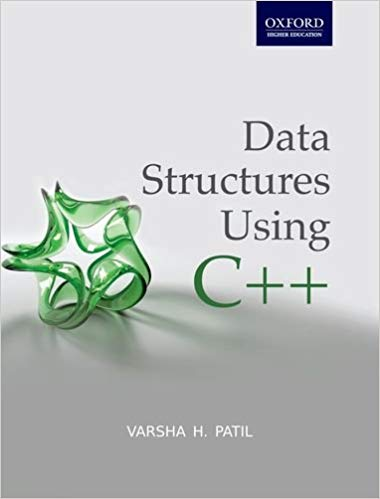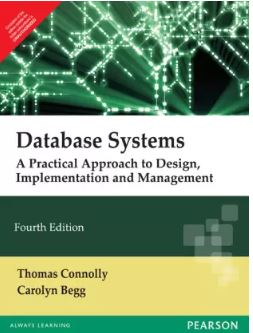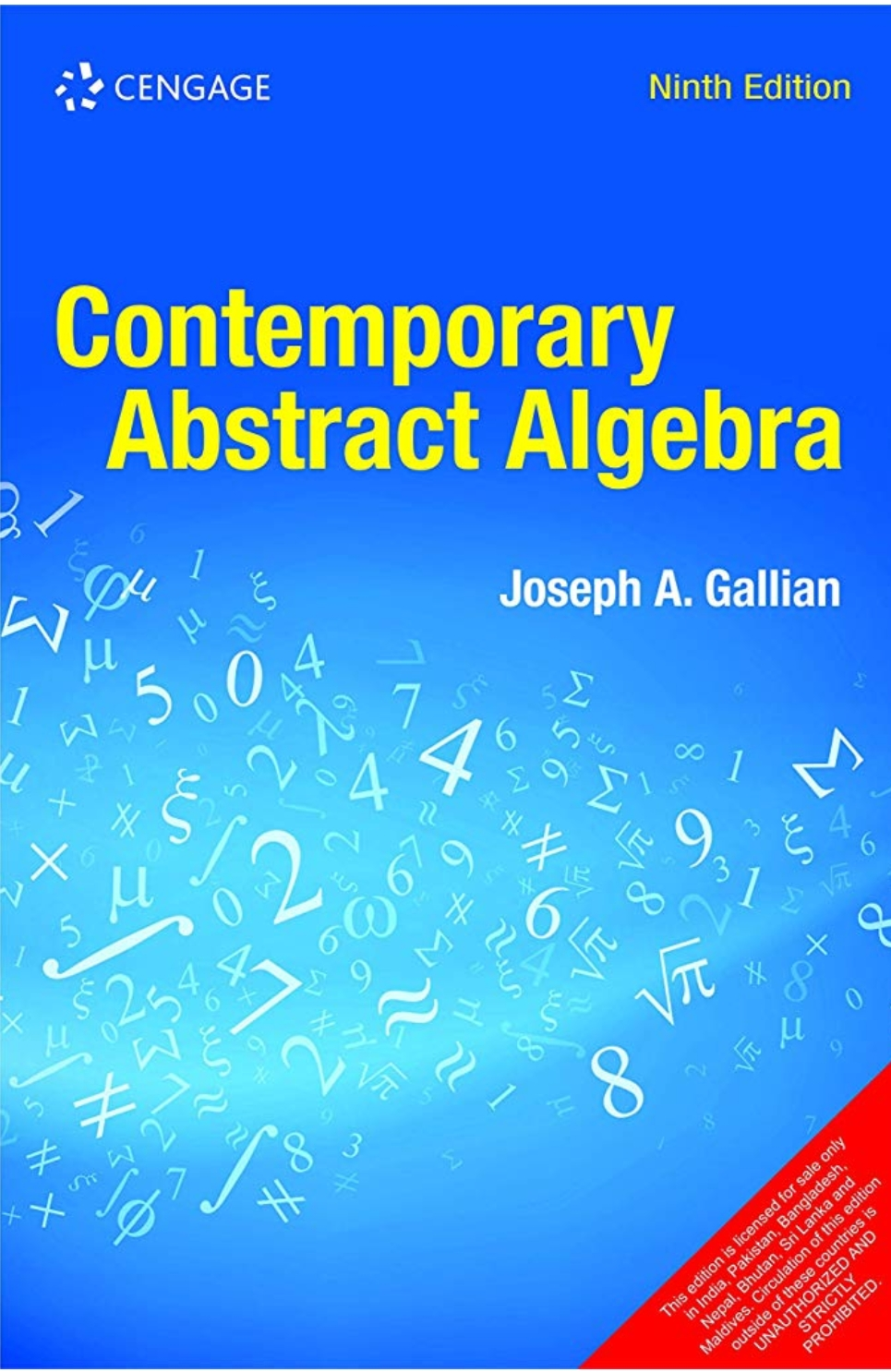### Computer Science - III

Course Objectives

1. To impart the basic concepts of data structures and algorithms
2. To understanding about writing algorithms and step by step approach in solving problems with the help of fundamental data structures
3. To understand the abstract data types stack, queue, deque, list, trees and graphs.
4. To be able to implement the ADTs stack, queue, and deque using the C++ programming language.
5. To understand the performance of the implementations of basic linear data structures.
6. To understand prefix, infix, and postfix expression formats.
7. To use stacks to evaluate postfix expressions.
8. To use stacks to convert expressions from infix to postfix.
9. To be able to implement the abstract data type list as a tree or graph using the node and reference pattern.
10. To understand concepts about searching and sorting techniquesData structure is logical or mathematical organization of data.

Course Outcomes

It describes how to store the data and access data from memory. To efficiently manage memory we required data structure.

CO1 - Students develop knowledge of basic data structures for storage and retrieval of ordered or unordered data.Data structures include: arrays, linked lists, binary trees, heaps, and hash tables.

CO2 - Students develop knowledge of applications of data structures including the ability to implement algorithms for the creation, insertion, deletion, searching, and sorting of each data structure.

CO3 -  Students learn to analyze and compare algorithms for efficiency using Big-O notation.

CO4 - Ability to analyze algorithms and algorithm correctness.

CO5 - Ability to describe stack,queue and linked list operation.

CO6Students will be able to use linear and non-linear data structures like stacks, queues linked list,tree and graphs.

CO7 Ability to summarize searching and sorting techniques.

CO8 Describe the hash function and concepts of collision and its resolution methods

CO9 Student will be able to choose appropriate data structure as applied to specified problem definition.### Computer Science -IV(Database Management Systems)

Course Objective:

The objective of the course is to present an introduction to database management systems, with an emphasis on how to organize, maintain and retrieve information from a DBMS efficiently and effectively.

Course Outcome:-

By the end of the course, the student should be able to:

CO1. Understand a database system

CO2. Understand relational model of the data

CO3. Understand query languages for databases

CO4. Understand the role of the database administrator.

CO5. Competency for implementing a small Database system.

CO6.  Be proficient in SQL and PL/SQL

CO7.Understand basic transaction processing concepts

CO8. Understand different database recovery and security methods### Algebra, Mathematics, Paper - IV